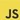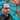# Common Higher Order Array Methods

September 23, 2019

Common higher order functions(HOF) are map, filter and reduce amongst others and these are the one’s we are going to see in this tutorial. First we are going to see how each of them work and after that we are going to combine them.

##Prerequisites:

• Basic knowledge of Javascript

Paraphrasing Wikipedia’s definition:

A higher-order function is a function that either takes a function as an argument or returns a function as a result.

Now let’s see some examples.

##map()

With map() we perform an action in every element of the array. Let say I have an array of numbers.

``let arr = [1, 2, 3, 4, 5]``

I can return each number multiplied by two like so.

``const numbers = arr.map(a => a * 2)``

a is each element of the array in every iteration. This will return …

``;[2, 4, 6, 8, 10]``

##filter()

filter() creates a new array based on if a condition is true or false for each element. If true the element will be added to the new array, if false it won’t be added. For example if I want a new array with only the even numbers of the initial array, I’ll have to check each number if divided by two doesn’t leave us a remainder. That means it’s an even number.

``const evenNumbers = arr.filter(a => a % 2 === 0)``

The above will return.

``;[2, 4]``

##reduce()

reduce() executes a function on every element resulting in a single value. It needs at least two parameters. The accumulator and the currentValue. As the name implies the accumulator accumulates the values returned from each iteration. The currentValue is the current element being processed.

We can find the sum of all the numbers like so.

``const sumNumbers = arr.reduce((acc, curr) => acc + curr)``

The above will return.

``15``

So what is basically happening here?

• 1st iteration the curr is 1 and the acc 1
• 2nd iteration the curr is 2 and the acc 3 (1 + 2)
• 3rd iterations the curr is 3 and the acc is 6 (1 + 2 + 3) and so on …

##Combining array methods

Combining array methods you can see the true power of javascript. Here is an example using map(), filter() and reduce() all together.

``````const number = arr
.filter(a => a % 2 === 0)
.map(a => a * 2)
.reduce((acc, curr) => acc + curr)``````

The above will return.

``12``

Hope this was of some value. For more array methods I have another article as well.In love with JavaScript, React and programming fundamentals in general.